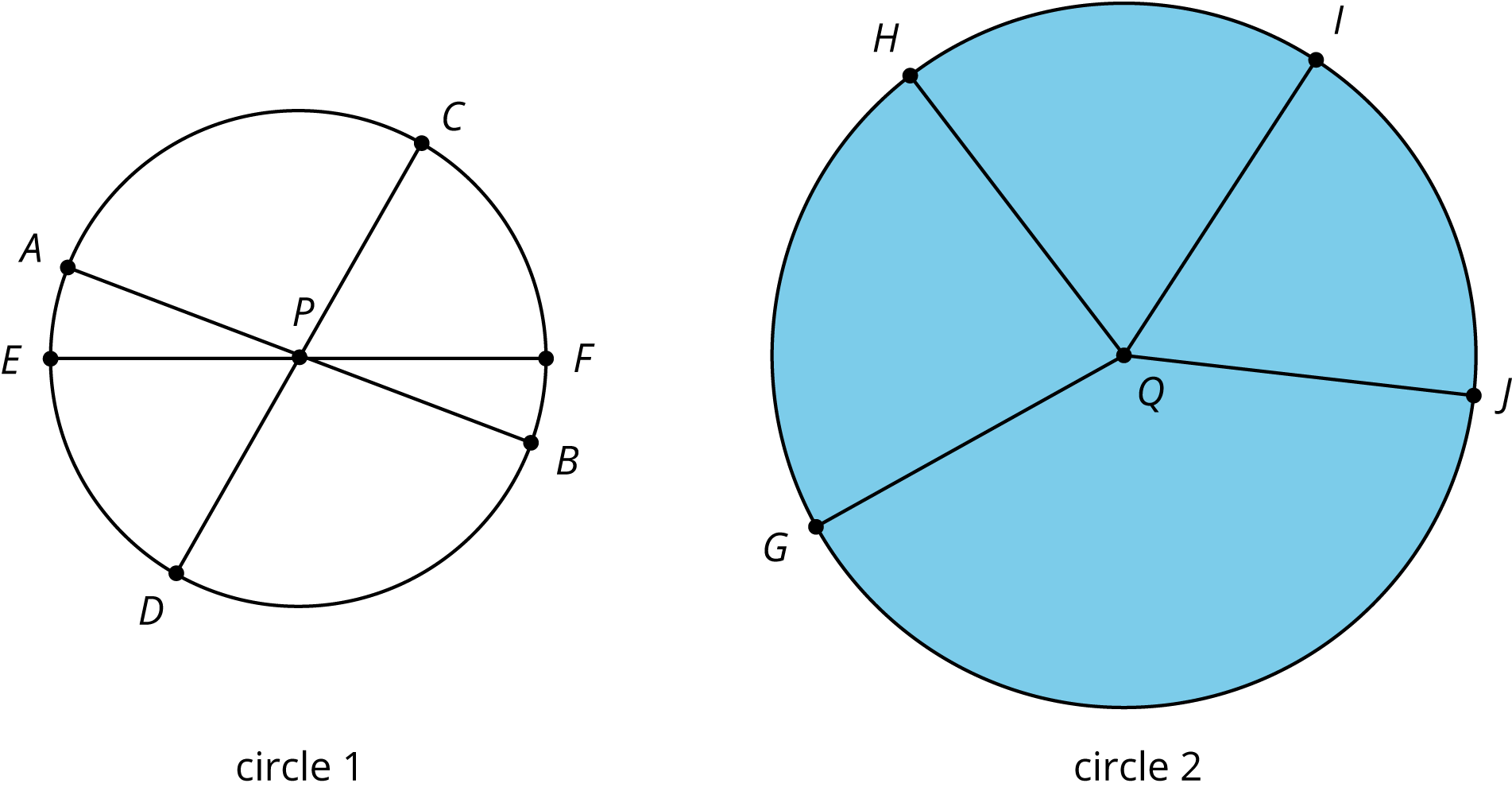## Circumference of a Circle

This week your student will learn why circles are different from other shapes, such as triangles and squares. Circles are perfectly round because they are made up of all the points that are the same distance away from a center.• This line segment from the center to a point on the circle is called the radius. For example, the segment from P to F is a radius of circle 1.
• The line segment between two points on the circle and through the center is called the diameter. It is twice the length of the radius. For example, the segment from E to F is a diameter of circle 1. Notice how segment EF is twice as long as segment PF.
• The distance around a circle is called the circumference. It is a little more than 3 times the length of the diameter. The exact relationship is $C=\pi d$, where $\pi$ is a constant with infinitely many digits after the decimal point. One common approximation for $\pi$ is 3.14.

We can use the proportional relationships between radius, diameter, and circumference to solve problems.

Here is a task to try with your student:

A cereal bowl has a diameter of 16 centimeters.

1. What is the radius of the cereal bowl?
1. 5 centimeters
2. 8 centimeters
3. 32 centimeters
4. 50 centimeters
2. What is the circumference of the cereal bowl?
1. 5 centimeters
2. 8 centimeters
3. 32 centimeters
4. 50 centimeters

Solution:

1. B, 8 centimeters. The diameter of a circle is twice the length of the radius, so the radius is half the length of the diameter. We can divide the diameter by 2 to find the radius. $16 \div 2 = 8$.
2. D, 50 centimeters. The circumference of a circle is $\pi$ times the diameter. $16 \boldcdot 3.14 \approx 50$.

## Area of a Circle

This week your student will solve problems about the area inside circles. We can cut a circle into wedges and rearrange the pieces without changing the area of the shape. The smaller we cut the wedges, the more the rearranged shape looks like a parallelogram.The area of a circle can be found by multiplying half of the circumference times the radius. Using $C=2\pi r$ we can represent this relationship with the equation: $$A = \frac12 (2\pi r) \boldcdot r$$ Or $$A=\pi r^2$$ This means that if we know the radius, we can find the area. For example, if a circle has radius 10 cm, then the area is about 314 cm2, because $3.14 \boldcdot 10^2 = 314$. We can also say that the area is $100\pi$ cm2.

Here is a task to try with your student:

A rectangular wooden board, 20 inches wide and 40 inches long, has a circular hole cut out of it.

1. The diameter of the circle is 6 inches. What is the area?
2. What is the area of the board after the circle is removed?

Solution:

1. $9\pi$ or about 28.26 in2. The radius of the hole is half of the diameter, so we can divide $6 \div 2 = 3$. The area of a circle can be calculated $A = \pi r^2$. For a radius of 3, we get $3^2=9$. We can write $9\pi$ or use 3.14 as an approximation of pi, $3.14 \boldcdot 9 = 28.26$.
2. $800 - 9\pi$ or about 771.74 in2. Before the hole was cut out, the entire board had an area of $20 \boldcdot 40$ or 800 in2. We can subtract the area of the missing part to get the area of the remaining board, $800 - 28.26 = 771.74$.